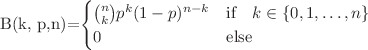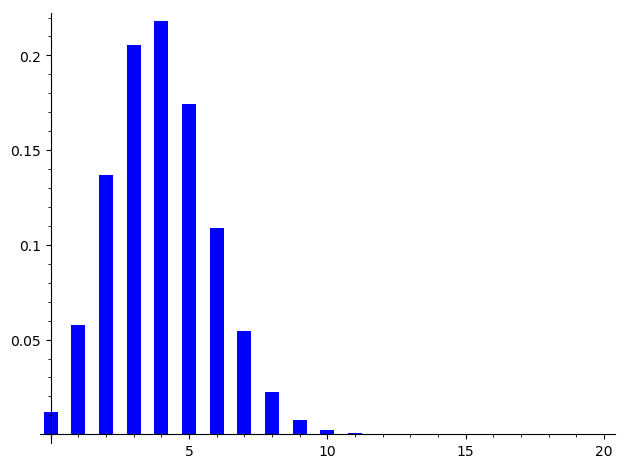# How to plot the Binomial Distribution

Hey there, is there a macro for?

edit retag close merge delete

Sort by » oldest newest most voted

The formula can be written in SageMath using binomial, but you may also use binom from the scipy library:

sage: import scipy.stats
sage: binom_dist = scipy.stats.binom(20,.20)
sage: bar_chart([binom_dist.pmf(k) for k in range(20)])See the Sage quick start for statistics page. As explained in the doc of

sage: scipy.stats.binom?


pmf stands for Probability mass function.

more